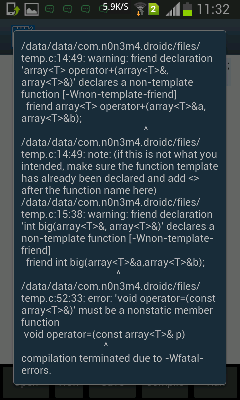#include ＜iostream＞
using namespace std;
template ＜typename T＞
class array
{
private:
T *A;
int L;
public:
array(int a);
array();
array(array＜T＞ &);
void datain(T a);
friend array＜T＞ operator+(array＜T＞&a,array＜T＞&b);
friend int big(array＜T＞&a,array＜T＞&b);
void operator=(const array＜T＞&);
void show();
T average();
~array();
};
template ＜typename T＞
array＜T＞::array(int a)
{
int c=0;
A=new T[a];
while (c＜a)
{
cin＞＞A[c];
c++;
}
L=a;
}
template ＜typename T＞
array＜T＞::array()
{
A=NULL; L=0;
}
template ＜typename T＞
array＜T＞::~array()
{
delete []A;
}
template ＜typename T＞
array＜T＞::array(array＜T＞ &p)
{
L=p.L;
A=new T[L];
for (int j=0;j＜L;j++)
A[j]=p.A[j];
}
template ＜typename T＞
void operator=(const array＜T＞& p)
{
L=p.L;
A=new T[L];
for (int j=0;j＜L;j++)
A[j]=p.A[j];
}
template ＜typename T＞
void array＜T＞::datain(T a)
{
A[L-1]=a;
}
template ＜typename T＞
friend int big(array＜T＞&a,array＜T＞ &b)
{
if (a.L＞=b.L)
return a.L;
else
return b.L;
}
template ＜typename T＞
friend array＜T＞ operator+(array＜T＞&a,array＜T＞&b)
{
array＜T＞ c;
int BIG=big(a,b);
c.L=BIG;
c.A=new T[BIG];
for (int i=0;i＜BIG;i++)
{
c.A[i]=a.A[i]+b.A[i];
}
return c;
}
template ＜typename T＞
T array＜T＞::average()
{
T all=0;
for (int i=0;i＜L;i++)
{
all+=A[i];
}
return all/L;
}
template ＜typename T＞
void array＜T＞::show()
{
for (int i=0;i＜L;i++)
cout＜＜A[i]＜＜" ";
cout＜＜endl;
}
int main()
{
array＜int＞ A1(3);
A1.show();
A1.datain(6);
A1.show();
cout＜＜A1.average()＜＜endl;
array＜int＞ B(3);
array＜int＞ C;
C=A1+B;
C.show();
return 0;
}0

3个回答

1.所有的<>，<<符号都打错了，可以按Shift+space转换下。
2.友元函数在类外实现的时候不用加friend。
3.“=”赋值运算符重载实现的时候没有加“array::”。
4.重载的+这个函数若是两个长度不相等的array相加就会造成越界，假设b.L为3，a.L为2，那么for循环里面最后会出现c.A=a.A+b.A,显然a.A这个数组根本没有这个下标，它最多为a.A。可以用两个循环给要返回的数组赋值，先赋值两个都有元素的位置，再复制另一个里面多余的元素到相应的位置。

0Crazy_Plus 回复YXTS122: 不客气
2 年多之前 回复YXTS122 template＜T＞friend array＜T＞ operator++(array＜T＞&,array＜T＞&); 刚刚试着改成这样，果然可以运行了，谢谢了！
2 年多之前 回复Crazy_Plus 回复YXTS122: 哦，那问题解决了吧
2 年多之前 回复YXTS122 ＜＞＜＜这些符号我是特意写成中文下的，我知道要写成英文下。因为用手机提问，所以得这样写，要不然＜＞这些符号显示不出来
2 年多之前 回复Crazy_Plus 你的big函数里面的if (a.L＞=b.L)，>和=中间多了个空格！，还有模板类中的友元函数前面要加上template<typename T>,这样就解决所有的问题了
2 年多之前 回复Crazy_Plus 第三条那里应该是array<T>::
2 年多之前 回复
0
`````` #include<iostream>
using namespace std;
template <typename T>
class array
{
public:
T *A;
int L;
array(int a);
array();
array(array<T> &);
void datain(T a);
template<T> friend array<T> operator+(array<T>&a,array<T>&b);
template<T>friend int big(array<T>&a,array<T>&b);
void operator=(const array<T>&);
void show();
void average();
~array();
};
template <typename T>
array<T>::array(int a)
{
int c=0;
A=new T[a];
while (c<a)
{
cin>>A[c];
c++;
}
L=a;
}
template <typename T>
array<T>::array()
{
A=NULL;   L=0;
}
template <typename T>
array<T>::~array()
{
delete []A;
}
template <typename T>
array<T>::array(array<T> &p)
{
L=p.L;
A=new T[L];
for (int j=0;j<L;j++)
A[j]=p.A[j];
}
template <typename T>
void array<T>::operator=(const array<T>& p)
{
L=p.L;
A=new T[L];
for (int j=0;j<L;j++)
A[j]=p.A[j];
}
template <typename T>
void array<T>::datain(T a)
{
A[L-1]=a;
}
template <typename T>
int big(array<T>&a,array<T>&b)
{
if (a.L>=b.L)
return a.L;
else
return b.L;
}
template <typename T>
array<T> operator+(array<T>&a,array<T>&b)
{
int i,x;
array<T>  c;
int BIG=big(a,b);
c.L=BIG;
c.A=new T[BIG];
if (a.L<b.L)
x=a.L;
else if (a.L>=b.L)
x=b.L;
for (i=0;i<x;i++)
{
c.A[i]=a.A[i]+b.A[i];
}
if (a.L<b.L)
for (;i<b.L;i++)
c.A[i]=b.A[i];
else if (a.L>=b.L)
for (;i<a.L;i++)
c.A[i]=a.A[i];
return c;
}
template <typename T>
void  array<T>::average()
{
T all=0;
for (int i=0;i<L;i++)
{
all+=A[i];
}
cout<<all/L<<endl;
}
template <typename T>
void array<T>::show()
{
for (int i=0;i<L;i++)
cout<<A[i]<<" ";
cout<<endl;
}
int main()
{
array<int>A1(3);
A1.show();
A1.datain(6);
A1.show();
A1.average();
array<int>B(2);
array<int>  C;
C=A1+B;
C.show();
return 0;
}
``````
0birt报表参数日期选择JS文件及操作步骤

Revit二次开发--数据库事件
Revit二次开发--数据库事件。改正了书上的一些错误
java计算器（修订）

C# 改错系统

rational rose Debug
rose2003 出现的一些错误，对于错误改正，需要用到的一件附件下载。
C++采用梯形法和辛普生法求定积分
C++设计采用梯形法和辛普生法求定积分,文档中附带代码,有需要的同学可以下载学习.
java编程运行错误集
java编程中使用hashmap时常见错误及改正

c++宝贵错误与改正
c++宝贵错误与改正
C语言两个正整数的最小公倍数

CSerialPort类，支持unicode编码

cass房檐改正插件

java 应用开发jar包

android简易画图工具

json_jar包

python下载资源

pMachine v2.3汉化版

MySql常见错误表

oracle常见错误及解决方法
oracle常见错误代码，如ORA-XXXX类型的错误及其解决方法

Cognos C10 Linux安装时 缺失的三个包

20140927Eclipse错误日志
20140927Eclipse错误日志
ccs调试中报错
ccs在使用过程中可能会出现的错误以及相应的改正方法。
C#串口提取ＧＰＳ数据

c/c++面试题大全

cass 自定义符号库完整教程
cass 帮助文件的自定义符号教程。内部有错误，这里将错误改正，别做好详细教程